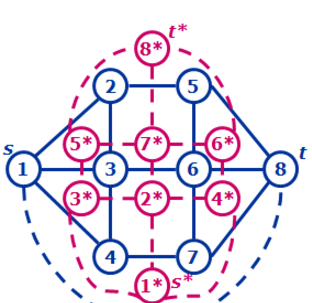LOJ上的模板题

LOJ上的模板题

LOJ上的模板题

# 最小割

## 平面图最小割# 最大权闭合图

$A=A^++A^-=A^++B^+-(B^+-A^-)=V^+-cut(G')$

# 最大密度图

$\max \phantom{1}\frac{|E'|}{|V'|}$

$h(c)=max\{|E'|-c|V'|\Big|G'=(V',E')\subseteq G\}$

$\frac{|E_A|}{|V_A|}-\frac{|E_B|}{|V_B|}=\frac{|E_A||V_B|-|E_B||V_A|}{|V_A||V_B|}\geq \frac{1}{|V_A||V_B|}\geq \frac{1}{|V|^2}$

$\log_2{\frac{|E|-0}{\frac{1}{|V|^2}}}=O(\log_2|V|)$

# 最小费用修复网络问题

• $\forall 1\leq i\leq n(0\leq f'_i\leq c'_i)$
• 每个顶点的入流和出流相等

• 从$u_i$到$v_i$连一条容量为$\max(c_i-f_i,0)$的费用为$a_i$的边。
• 从$v_i$到$u_i$连一条容量为$\min(f_i,c_i)$的费用为$a_i$的边。
• 从$u_i$到$v_i$连一条容量为无穷，费用为$a_i+b_i$的边。
• 从$v_i$到$u_i$连一条容量为$\max(f_i-c_i,0)$的费用为$a_i-b_i$的边。

• $\forall 1\leq i\leq n(0\leq f'_i\leq c'_i)$
• 除了源点和汇点外，每个顶点的入流和出流相等